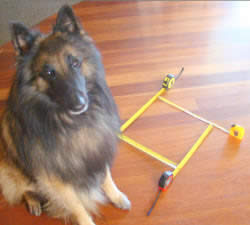# US Standard Area

These are the most common measurements:

• Square Inch
• Square Foot
• Square Yard
• Acre
• Square Mile

Small surfaces are usually measured in square inches, rooms and buildings in square feet, carpet in square yards, property in acres, and territory in square miles.

## Square InchArea is length by length, so a square inch is a square that is 1 inch on each side.

The Unit is inch × inch, which can be written many ways, such as:

• in2
• inch2
• sq in
• sq inch
• square inch
• etc ...

## Square FootA square foot is a square that is 1 foot on each side.

Here Ariel the Dog sits next to a square foot made with tape measures.

The Unit is foot × foot, which can be written many ways, such as

• ft2
• foot2
• sq ft
• sq foot
• sq feet

Thinking of houses, the area of a typical ...... bedroom is 100 square feet
... garage is 400 square feet
... house is 2000 square feet

## Square Yard

A square yard is a square that is 1 yard on each side. It is equal to 9 square feet.

## AcreOne acre is equal to 43,560 square feet.

It is:

• a bt more than 200 ft by 200 ft
• exactly 198 ft by 220 ft
• exactly 66 ft by 660 ft
• about the area of a football field

Acres are commonly used to measure land.

## Square Mile

A square mile is a square that is 1 mile on each side.

Square miles are commonly used to measure large areas of land.

## More Examples

• 6½ square centimeters

An acre is:

• 4,840 square yards
• 43,560 square feet
• 4,046.8564224 square meters
• about 0.4 hectare (40% of the area of a hectare)
• a square with sides about 208.71 feet (or 69.57 yards)
• a bit less than a football field